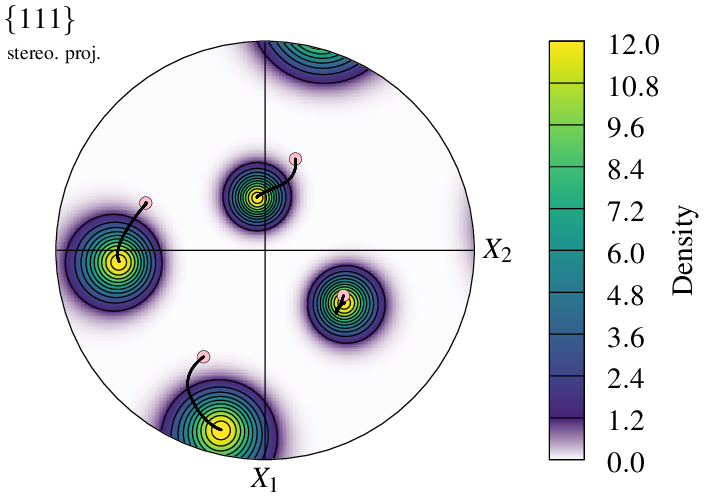# Plotting a Pole Figure

Important

A pole figure can be generated using the Visualization Module (-V), by switching to pole figure space, using `-space` `pf`. Pole figures can be generated from one or several inputs, among those of the Visualization Module (-V), such as the orientations of a tessellation cells, an orientation file and/or a direction file, making it possible to produce advanced figures.

Visualization in pole figure space works in the same way as visualization in standard, real space: the entity properties can be specified using the `-data*` options, the entities to show can be specified using the `-show*` options, etc. A difference is that the list of entities reduces to `cell` if the input is a tessellation, and `<input>` (or the default `point`) if the input is a custom input (orientations or directions).

In the following, and unless mentionned otherwise, a simple tessellation is used:

```# \$ neper -T -n 100 -morpho gg -oricrysym cubic -o n100
```

The tessellation can be visualized as follows:

```\$ neper -V n100.tess -imagesize 800:400 -print img1
```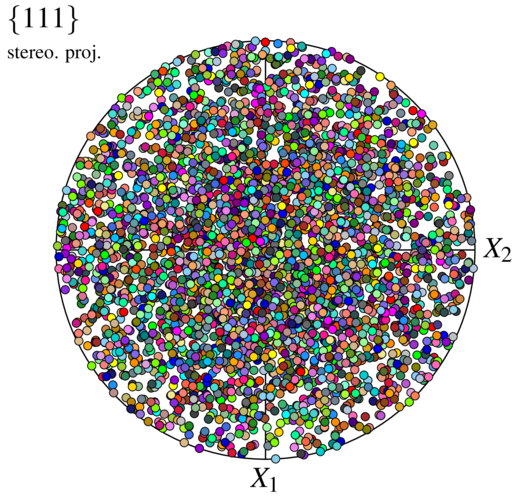To reproduce exactly the images below, add the following line to your `\$HOME/.neperrc` file (or to a local configuration file to be loaded with `--rcfile`):

```neper -V -imagesize 500:500
```

## Generalities

The tessellation orientations can be visualized using `-space` `pf`:

```\$ neper -V n100.tess -space pf -print img2
```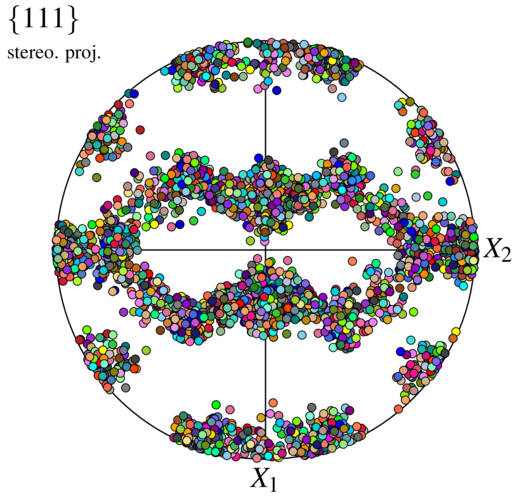This generates a PNG image named `img2.png`. By default, a pole figure is plotted from the (four) {111} poles, using a stereographic projection in the 1–2 reference plane, and all cell orientations/poles are represented using symbols. Orientations are colored from their (cell) ids, so that colors match between visualizations in real space an pole figure space (the two images above).

Note

Even if only orientations/poles are represented in pole figure space, `cell` is used to represent a cell orientation, which makes it possible to use the same options, `-showcell`, `-datacellcol`, etc. in all spaces.

For pole figures, it is also possible to write the output file at a vectorial PDF format:

```\$ neper -V n100.tess -space pf -imageformat pdf -print img2
```

`img2.pdf`

Note

The PDF format, as a vectorial format, provides an optimal quality/weight ratio and should be used when possible (for example, in LaTeX documents).

The pole family can be modified using `-pfpole`:

```\$ neper -V n100.tess -space pf -pfpole 1:0:0 -print img3
```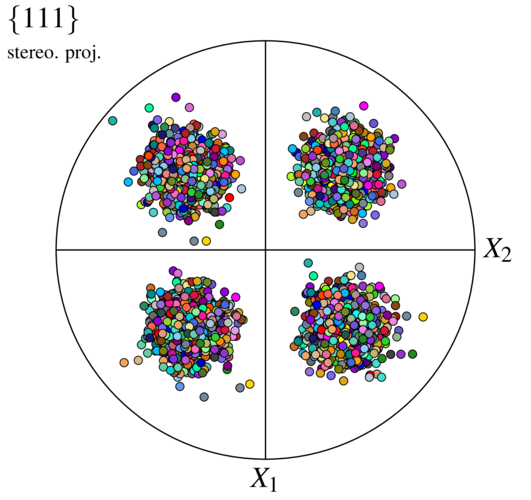The projection type can be modified using `-pfprojection`:

```\$ neper -V n100.tess -space pf -pfprojection equal-area -print img4
```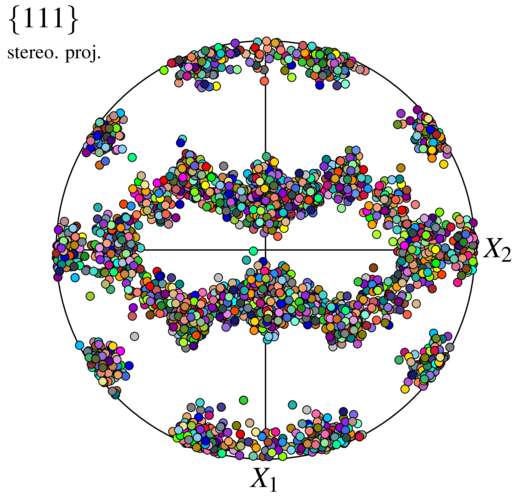The sample directions can be modified using `-pfdir`, so as the actual axis labels, using `-datacsyslabel`:

```\$ neper -V n100.tess -space pf -pfdir y:z -datacsyslabel RD:TD:ND -print img5
```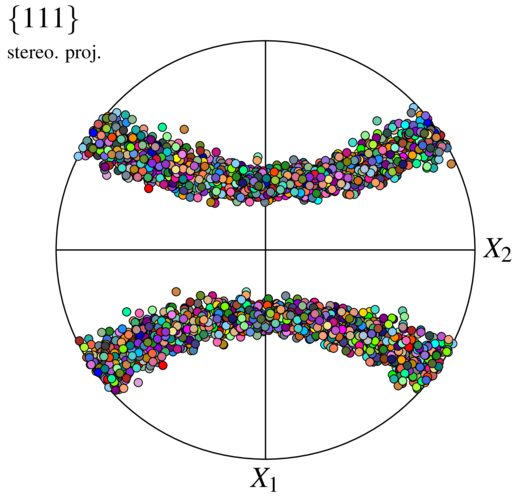The representation mode can be changed into `density`:

```\$ neper -V n100.tess -space pf -pfmode density -print img6
```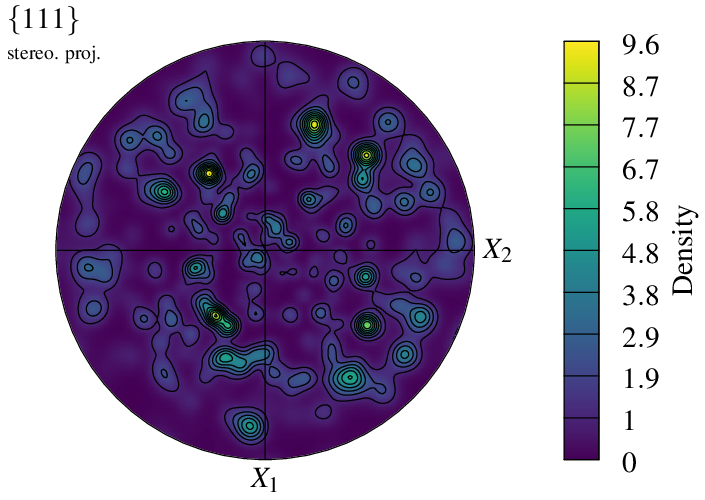It is even possible to superimpose the `symbol` and `density` representation modes:

```\$ neper -V n100.tess -space pf        \
-pfmode density,symbol        \
-datacellcol orange           \
-print img7
```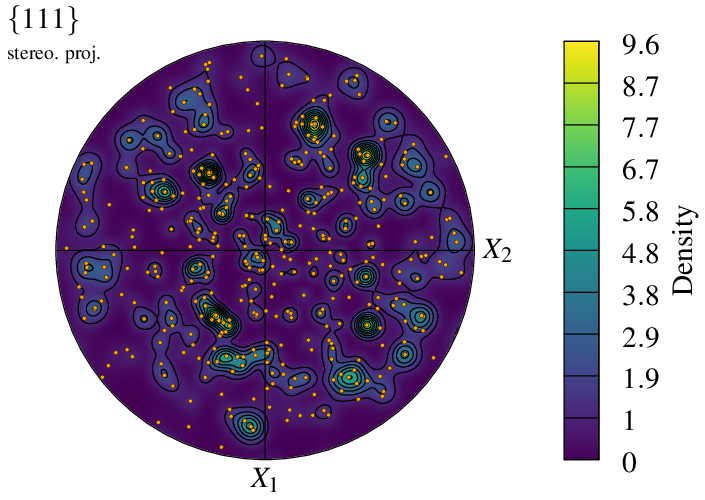The visualization can be reduced to a selection of cells using `-showcell` (as in real space). For instance, to show only the cells larger than the average:

```\$ neper -V n100.tess -space pf -showcell "vol>0.01" -print img8
```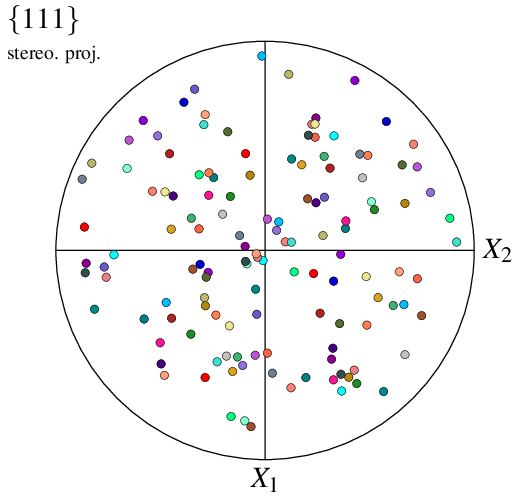Finally, it is possible to change the character font from the default `TimesRoman` to the LaTeX-style `ComputerModern`:

```\$ neper -V n100.tess -space pf -pffont ComputerModern -print img9
```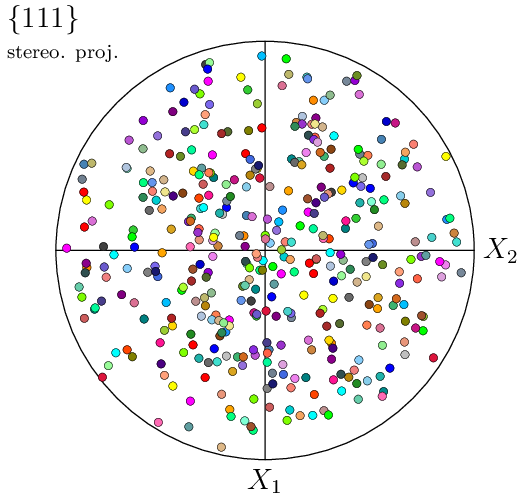## Configuring a Symbol Plot

For a tessellation, the way data are represented can be specified using options `-datacell*`. The properties include the color (options `-datacellcol` and `-datacellcolscheme`), transparency (option `-datacelltrs`), radius (or size) (option `-datacellrad`) and symbol (option `-datacellsymbol`).

The size of the poles can, for instance, be set to be proportional to the corresponding cell sizes, and their colors a function of the z cell positions:

```\$ neper -V n100.tess -space pf              \
-datacellcol z                      \
-datacellscale "0.0:1.0"            \
-datacellscaletitle "z position"    \
-print img10
\$ convert +append img10.png img10-scale3.png -alpha off img10.png
```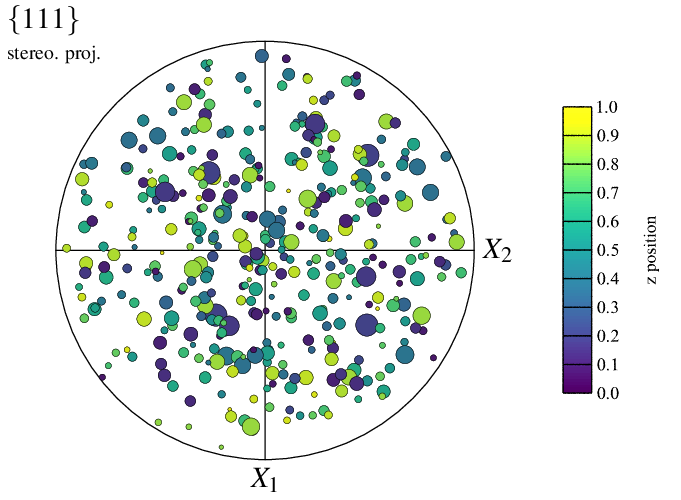This originally produces a PNG file named `img10.png` for the map and a PNG file named `img10-scale3.png` for the scale bar, which is pasted to `img10.png` thanks to convert.

The apparence of the symbol “edges” can also be specified, using options `-datacelledgerad` and `-datacelledgecol`. For example:

```\$ neper -V n100.tess -space pf                  \
-datacellcol z                                \
-datacellscale "0.0:1.0"                      \
-datacellscaletitle "z position"              \
-datacelledgecol orange                       \
-print img11
\$ convert +append img11.png img11-scale3.png -alpha off img11.png
```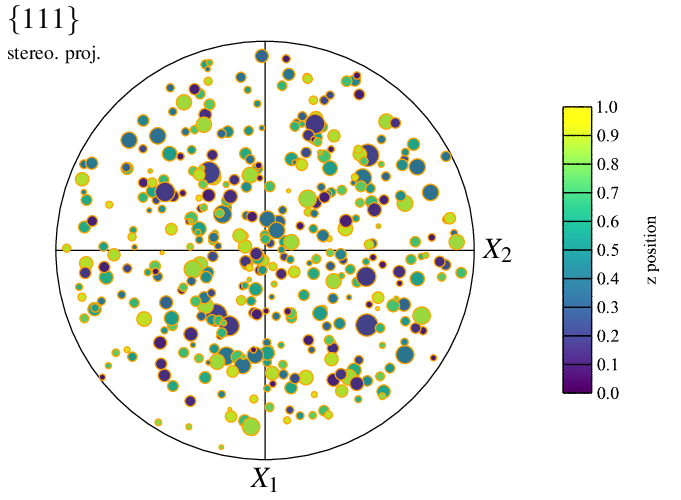The type of symbols can be specified using `-datacellsymbol`. For example, to use squares:

```\$ neper -V n100.tess -space pf                  \
-datacellsymbol "square"                      \
-print img12
```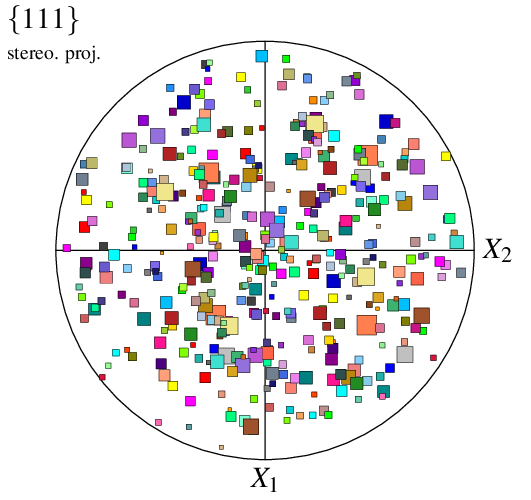When only the type of symbol is provided to `-datacellsymbol`, the radius is taken from option `-datacellrad` (either the provided value or the default value). Alternatively, values can be provided in `-datacellsymbol` itself, as well as an angle; different symbols can even be specified for the different cells, using a Data File (`n100-symbols`), to produce a fully customized plot:

```\$ neper -V n100.tess -space pf                 \
-datacellsymbol "file(n100-symbols)"         \
-print img13
```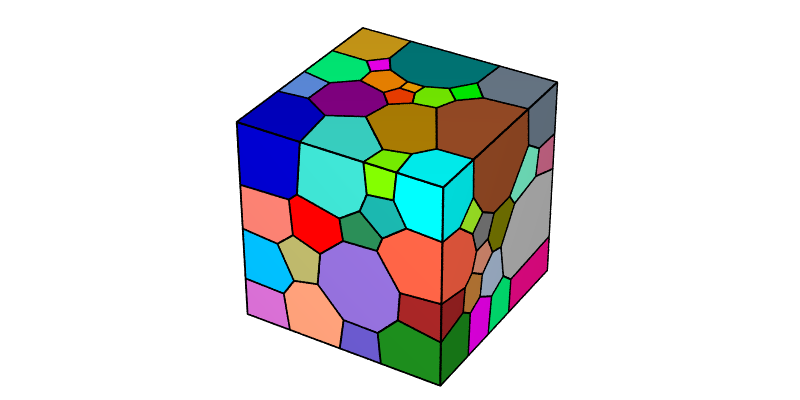## Configuring a Density Field Plot

A tessellation with typical rolling orientations is used in the following: `n200-psc.tess`.

The (default) symbol plot can be turned into a density field plot using `-pfmode` `density`.

```\$ neper -V n200-psc.tess -space pf            \
-pfmode density                    \
-print img14
```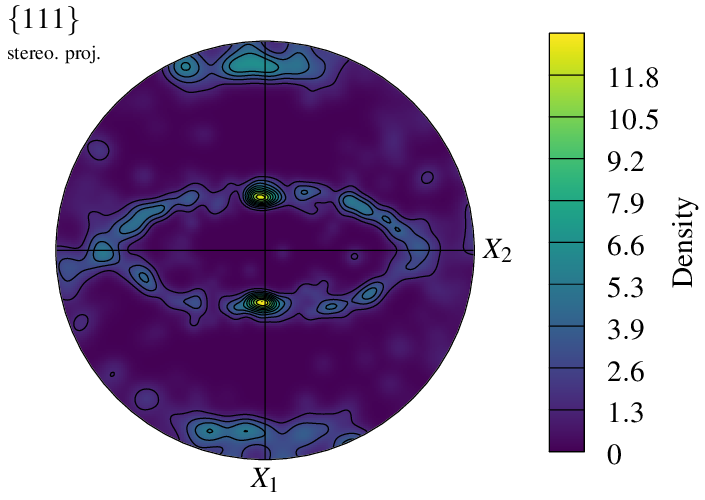A density plot is plotted with a color field and contour lines that correspond to the scale ticks.

The scale extent and ticks (and so the values of the contour lines) can be modified using `-datacellscale`:

```\$ neper -V n200-psc.tess -space pf            \
-pfmode density                    \
-datacellscale 0:2:4:6:8:10:12     \
-print img15
```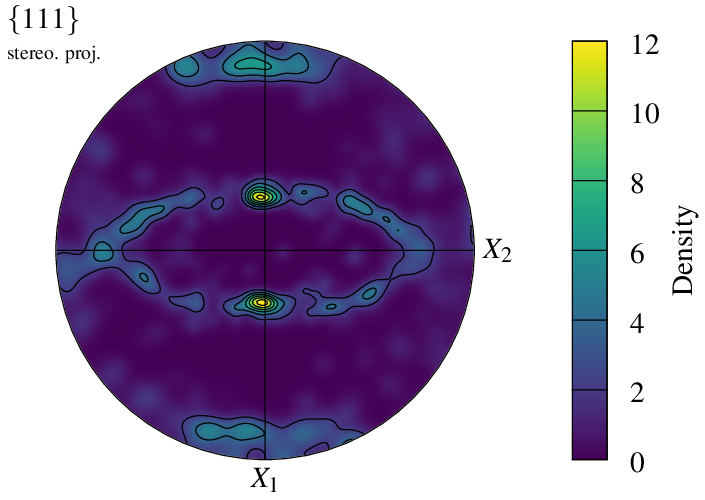A density field is computed by assigning a kernel to each pole, which is defined by `-pfkernel` (default `normal(theta=3)`). A different value changes the intensity of the density field:

```\$ neper -V n200-psc.tess -space pf            \
-pfmode density                    \
-pfkernel "normal(theta=5)"        \
-datacellscale 0:1:2:3:4:5:6       \
-print img16
```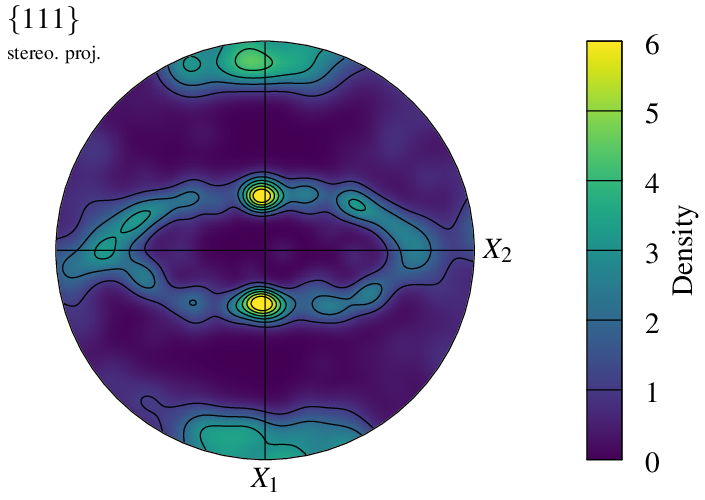Symmetry conditions in the reference coordinate system can be defined using `-pfsym` (default `monoclinic`). It can be set to `orthotropic`:

```\$ neper -V n200-psc.tess -space pf            \
-pfmode density                    \
-pfkernel "normal(theta=5)"        \
-pfsym orthotropic                 \
-datacellscale 0:1:2:3:4:5:6       \
-print img17
```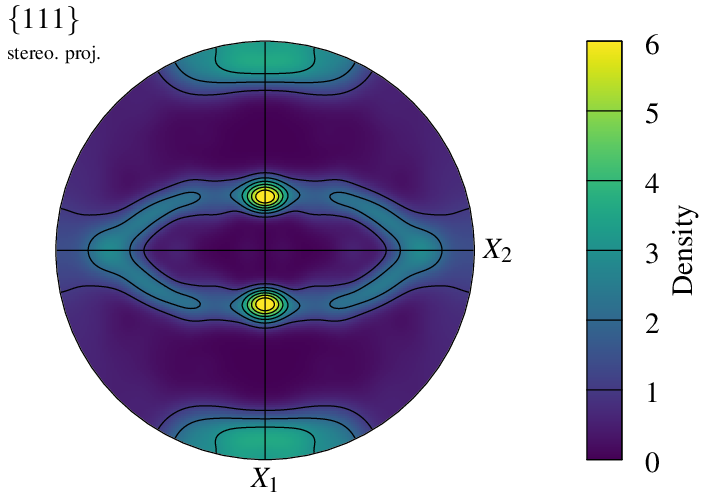In the case of orthotropic symmetry, the pole figure can be reduced to one quarter using `-pfsym` `quarter` (default `full`):

```\$ neper -V n200-psc.tess -space pf            \
-pfmode density                    \
-pfkernel "normal(theta=5)"        \
-pfsym orthotropic                 \
-pfshape quarter                   \
-datacellscale 0:1:2:3:4:5:6       \
-print img18
```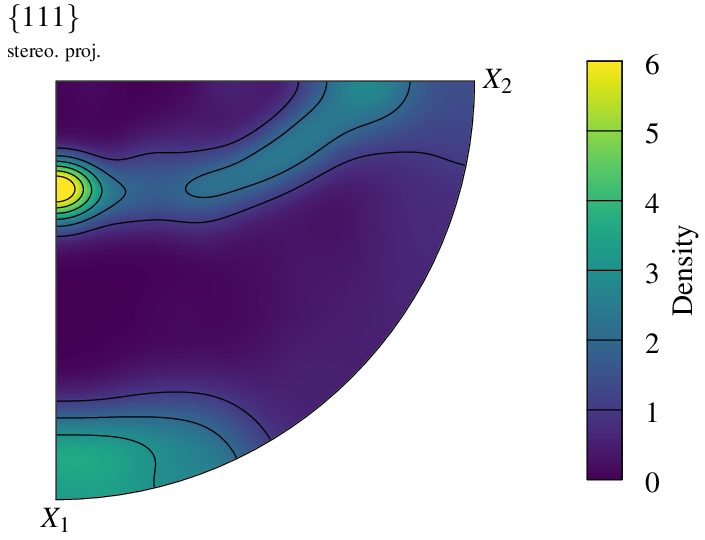The density field is defined on a regular grid, whose dimensions can be modified using `-pfgridsize`. While the default value of `200` provides a good balance between quality and computation time, the option can be used to speed up generation or, at the opposite, increase quality:

```\$ neper -V n200-psc.tess -space pf            \
-pfmode density                    \
-pfkernel "normal(theta=5)"        \
-datacellscale 0:1:2:3:4:5:6       \
-pfsym orthotropic                 \
-pfgridsize 30                     \
-print img19                       \
-pfgridsize 300                    \
-print img20
```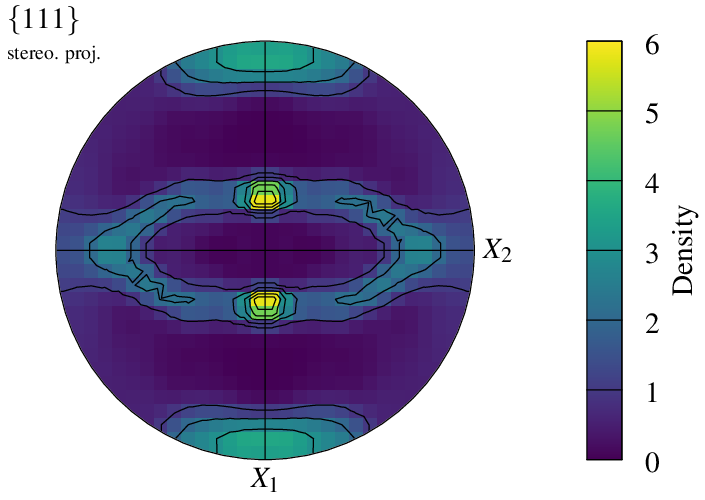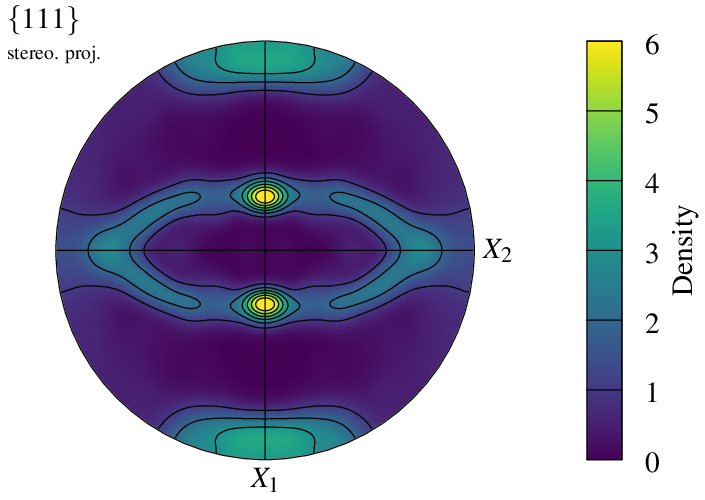It is possible to specify which color scheme to use for the density plot, using `-datacellcolscheme` (see Color Maps for Real Values):

```\$ neper -V n200-psc.tess -space pf            \
-pfmode density                    \
-pfkernel "normal(theta=5)"        \
-pfsym orthotropic                 \
-datacellscale 0.0:6               \
-print img21
```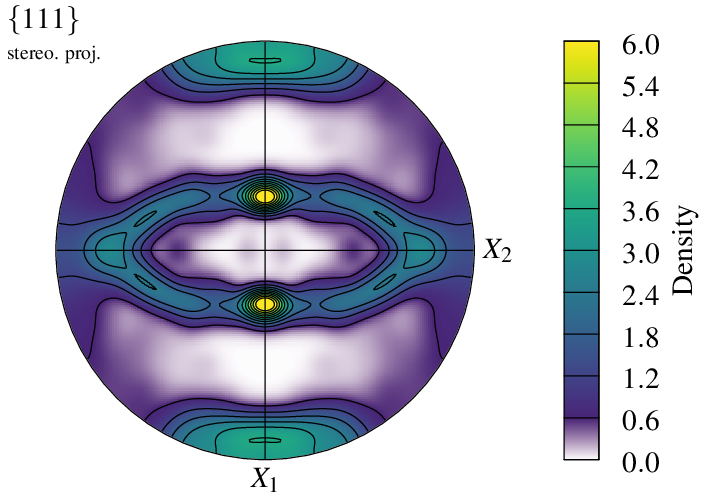## Working From an Orientation Input

A pole figure can be generated from an orientation file by loading it as a custom input (see Input Data). For instance, consider an orientation file, `n100.ori`, generated by Tessellation Module (-T):

```\$ neper -T -n 100 -for ori -o n100
```

The file can be loaded to the Visualization Module (-V) and plotted as a pole figure as follows:

```\$ neper -V n100.ori -space pf -print img22
```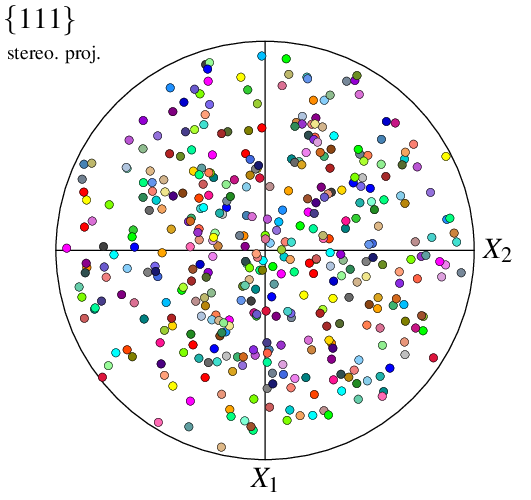Note

In `-space` `pf`, a custom input is interprated as an orientation file by default. The command produces the same image as if the orientations were read from a tessellation with the same orientations.

Since the orientations are not attached to cells, they are no longer called `cell` but `point` (by default) in options `-data*` and `-show*`. For example, to specify symbol radii:

```\$ neper -V n100.ori -space pf -datapointrad 0.01+0.03*id/100 -print img23
```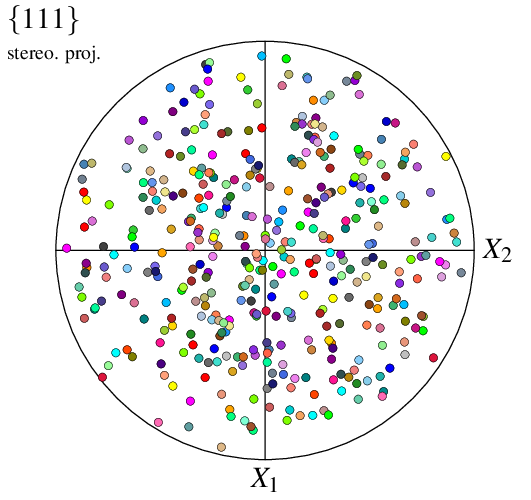## Working From a Direction Input

A pole figure can be created from directions (instead of orientations) loaded as a custom input (see Input Data). For instance, consider file `dir_file`. The file can be loaded to the Visualization Module (-V), as a direction file, and plotted as a pole figure as follows:

```\$ neper -V "mydir(type=vector):file(dir_file)" -space pf -print img24
```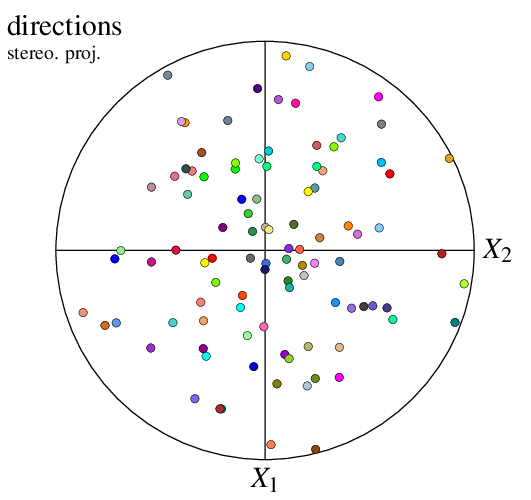The data are not called `point` anymore but `mydir` (or any other name defining the custom input), which defines new options, `-datamydir*` and `-showmydir`.

```\$ neper -V "mydir(type=vector):file(dir_file)" -space pf \
-print img25
```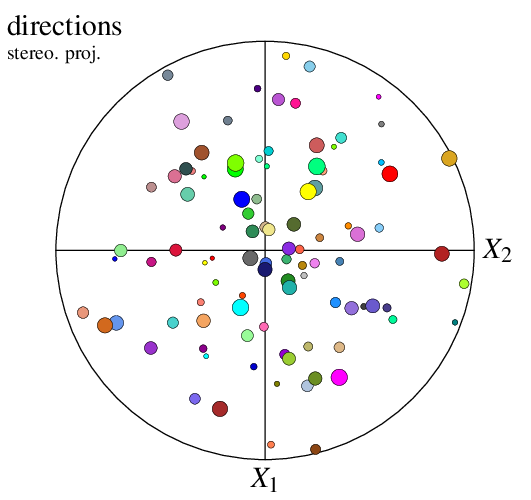## Working From Multiple Inputs

A pole figure can be created from multiple inputs loaded as custom inputs (see Input Data). This can, for example, be used to plot a (lattice) rotation path associated with plastic deformation. In the following, file `ini` contains an initial orientation, `all` its locations during plastic deformation, and `fin` the final orientation, all written as Rodrigues vectors.

The initial orientation, rotation path and final orientation can be visualized as follows:

```\$ neper -V "final(type=ori):file(fin),initial(type=ori):file(ini),path(type=ori):file(all)" \
-space pf                                     \
-print img26
```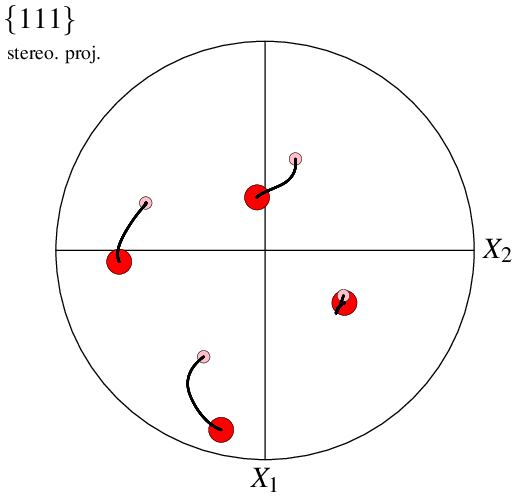It is also possible to plot in `density` mode, in which case the mode applies only to the first input. This can be used, for example, to mimic an orientation spread at final state:

```\$ neper -V "final(type=ori):file(fin),initial(type=ori):file(ini),path(type=ori):file(all)" \
-space pf                                     \